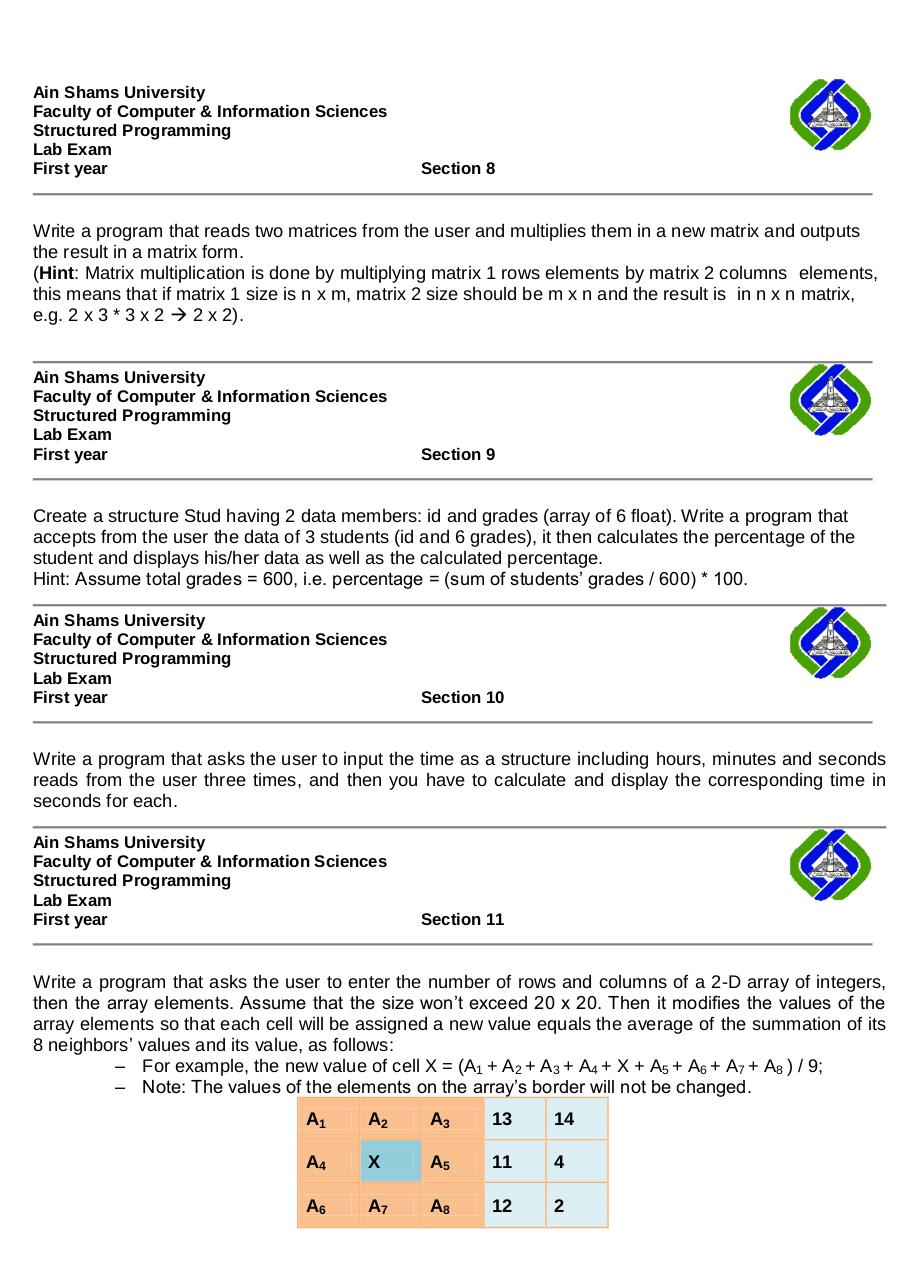# PDF Archive

Easily share your PDF documents with your contacts, on the Web and Social Networks.

## SP13 Lab 6 Quizzes.pdfPage 1 2 3 4 5 6

#### Text preview

Ain Shams University
Faculty of Computer &amp; Information Sciences
Structured Programming
Lab Exam
First year

Section 8

Write a program that reads two matrices from the user and multiplies them in a new matrix and outputs
the result in a matrix form.
(Hint: Matrix multiplication is done by multiplying matrix 1 rows elements by matrix 2 columns elements,
this means that if matrix 1 size is n x m, matrix 2 size should be m x n and the result is in n x n matrix,
e.g. 2 x 3 * 3 x 2  2 x 2).

Ain Shams University
Faculty of Computer &amp; Information Sciences
Structured Programming
Lab Exam
First year

Section 9

Create a structure Stud having 2 data members: id and grades (array of 6 float). Write a program that
accepts from the user the data of 3 students (id and 6 grades), it then calculates the percentage of the
student and displays his/her data as well as the calculated percentage.
Hint: Assume total grades = 600, i.e. percentage = (sum of students’ grades / 600) * 100.
Ain Shams University
Faculty of Computer &amp; Information Sciences
Structured Programming
Lab Exam
First year

Section 10

Write a program that asks the user to input the time as a structure including hours, minutes and seconds
reads from the user three times, and then you have to calculate and display the corresponding time in
seconds for each.
Ain Shams University
Faculty of Computer &amp; Information Sciences
Structured Programming
Lab Exam
First year

Section 11

Write a program that asks the user to enter the number of rows and columns of a 2-D array of integers,
then the array elements. Assume that the size won’t exceed 20 x 20. Then it modifies the values of the
array elements so that each cell will be assigned a new value equals the average of the summation of its
8 neighbors’ values and its value, as follows:
– For example, the new value of cell X = (A1 + A2 + A3 + A4 + X + A5 + A6 + A7 + A8 ) / 9;
– Note: The values of the elements on the array’s border will not be changed.
A1

A2

A3

13

14

A4

X

A5

11

4

A6

A7

A8

12

2